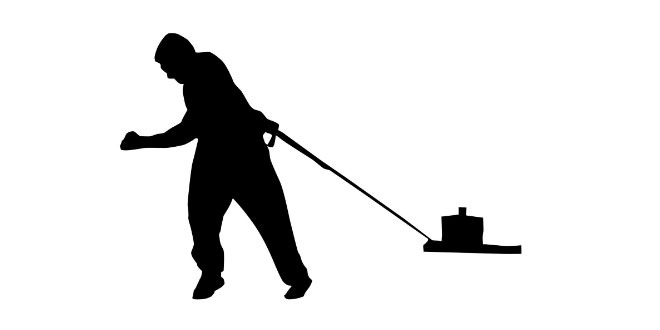Thursday , June 30 2022# NCERT 9th Class (CBSE) Science: Energy and Work

#### Question: State the law of conservation of energy.

Answer: It states that energy can neither be created nor destroyed. It can only change its form.

#### Question: In a tug-of-war one team gives way to the other. What work is being done and by whom?

Answer: The winning team does work. The work is equal to the product of the resultant force and the displacement undergone by the losing team.

#### Question: List two essential conditions for work to be done.

Answer: (i) A force must act and (ii) There should be displacement in the body.

#### Question: When is 1 joule of work said to be done?

Answer: When a force of 1 newton acting on a body displaces it in its own direction.

#### Question: What is power? What is its SI unit?

Answer: It is defined as the rate of doing work. Its unit is watt.

#### Question: Find the energy in kWh consumed in 10 hours by a machine of power 500 W.

Answer: W = P x t = 500 x 10 = 5000 Wh – 5 kWh.

#### Question:. When is work said to be done against the force of gravity ?

Answer: When a body lifted the work is done against the force of gravity.

#### Question: Write an expression for the work done in lifting a body of mass ‘m’ through a vertical height ‘h’.

Answer: Work done W = mgh, where g is acceleration due to gravity.

#### Question: When a book is lifted from a table, against which force work is done?

Answer: Work is done against the force of gravity.

#### Question: Is it possible that some force is acting on a body but still the work done is zero?

Answer: Yes, when force acts at an angle of 90° with the displacement.

#### Question: What is the work done on a body moving in a circular path?

Answer: Zero, because force and displacement are perpendicular to each other.

#### Question: A force of 7 N acts on an object. The displacement is, say 8 m, in the direction of the force. Let us take it that the force acts on the object through the displacement. What is the work done in this case?

Answer: Given, displacement = 8 m,
Force = 7N
Now, Work done = Force x Displacement
= 7 x 8 = 56 J

#### Question: When do we say that work is done?

Answer: Work is said to be done when a force causes displacement of an object in the direction of applied force.

#### Question: Write an expression for the work done when a force is acting on an object in the direction of its displacement.

Answer: Work done = Force x Displacement

#### Question: A pair of bullocks exert a force of 140 N on a plough. The field being ploughed is 15 m long. How much work is done in ploughing the length of the field ?

Answer: Work done = Force x Displacement = 140 x 15 = 2100 J

#### Question: What is the kinetic energy of an object?

Answer: The energy possessed by a body by virtue of its motion is called kinetic energy.

#### Question: Write an expression for the kinetic energy of an object.

Answer: The expression is KE = 1/2 mv2, where ‘m’ is the mass and V is the velocity of the body.

#### Question: Define 1 watt of power.

Answer: When a work of 1 joule is done in 1s, the power is said to be one watt.

## Sources of Energy: 10th Science Chapter 14

Class: 10th Class Subject: Science Chapter: Chapter 14: Sources of Energy Quiz: – Questions MCQs: …